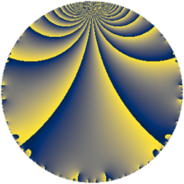# Properties

 Label 5610.2.a.gLevel $5610$ Weight $2$ Character orbit 5610.a Self dual yes Analytic conductor $44.796$ Analytic rank $0$ Dimension $1$ CM no Inner twists $1$

# Related objects

Show commands: Magma / PariGP / SageMath

## Newspace parameters

comment: Compute space of new eigenforms

[N,k,chi] = [5610,2,Mod(1,5610)]

mf = mfinit([N,k,chi],0)

lf = mfeigenbasis(mf)

from sage.modular.dirichlet import DirichletCharacter

H = DirichletGroup(5610, base_ring=CyclotomicField(2))

chi = DirichletCharacter(H, H._module([0, 0, 0, 0]))

N = Newforms(chi, 2, names="a")

//Please install CHIMP (https://github.com/edgarcosta/CHIMP) if you want to run this code

chi := DirichletCharacter("5610.1");

S:= CuspForms(chi, 2);

N := Newforms(S);

 Level: $$N$$ $$=$$ $$5610 = 2 \cdot 3 \cdot 5 \cdot 11 \cdot 17$$ Weight: $$k$$ $$=$$ $$2$$ Character orbit: $$[\chi]$$ $$=$$ 5610.a (trivial)

## Newform invariants

comment: select newform

sage: f = N # Warning: the index may be different

gp: f = lf \\ Warning: the index may be different

 Self dual: yes Analytic conductor: $$44.7960755339$$ Analytic rank: $$0$$ Dimension: $$1$$ Coefficient field: $$\mathbb{Q}$$ Coefficient ring: $$\mathbb{Z}$$ Coefficient ring index: $$1$$ Twist minimal: yes Fricke sign: $$-1$$ Sato-Tate group: $\mathrm{SU}(2)$

## $q$-expansion

comment: q-expansion

sage: f.q_expansion() # note that sage often uses an isomorphic number field

gp: mfcoefs(f, 20)

 $$f(q)$$ $$=$$ $$q - q^{2} - q^{3} + q^{4} + q^{5} + q^{6} - q^{8} + q^{9}+O(q^{10})$$ q - q^2 - q^3 + q^4 + q^5 + q^6 - q^8 + q^9 $$q - q^{2} - q^{3} + q^{4} + q^{5} + q^{6} - q^{8} + q^{9} - q^{10} - q^{11} - q^{12} + 2 q^{13} - q^{15} + q^{16} - q^{17} - q^{18} + 4 q^{19} + q^{20} + q^{22} + 8 q^{23} + q^{24} + q^{25} - 2 q^{26} - q^{27} + 6 q^{29} + q^{30} + 4 q^{31} - q^{32} + q^{33} + q^{34} + q^{36} - 6 q^{37} - 4 q^{38} - 2 q^{39} - q^{40} + 2 q^{41} - 8 q^{43} - q^{44} + q^{45} - 8 q^{46} - q^{48} - 7 q^{49} - q^{50} + q^{51} + 2 q^{52} + 2 q^{53} + q^{54} - q^{55} - 4 q^{57} - 6 q^{58} - q^{60} + 2 q^{61} - 4 q^{62} + q^{64} + 2 q^{65} - q^{66} - 4 q^{67} - q^{68} - 8 q^{69} + 8 q^{71} - q^{72} + 6 q^{73} + 6 q^{74} - q^{75} + 4 q^{76} + 2 q^{78} - 8 q^{79} + q^{80} + q^{81} - 2 q^{82} + 4 q^{83} - q^{85} + 8 q^{86} - 6 q^{87} + q^{88} + 2 q^{89} - q^{90} + 8 q^{92} - 4 q^{93} + 4 q^{95} + q^{96} - 10 q^{97} + 7 q^{98} - q^{99}+O(q^{100})$$ q - q^2 - q^3 + q^4 + q^5 + q^6 - q^8 + q^9 - q^10 - q^11 - q^12 + 2 * q^13 - q^15 + q^16 - q^17 - q^18 + 4 * q^19 + q^20 + q^22 + 8 * q^23 + q^24 + q^25 - 2 * q^26 - q^27 + 6 * q^29 + q^30 + 4 * q^31 - q^32 + q^33 + q^34 + q^36 - 6 * q^37 - 4 * q^38 - 2 * q^39 - q^40 + 2 * q^41 - 8 * q^43 - q^44 + q^45 - 8 * q^46 - q^48 - 7 * q^49 - q^50 + q^51 + 2 * q^52 + 2 * q^53 + q^54 - q^55 - 4 * q^57 - 6 * q^58 - q^60 + 2 * q^61 - 4 * q^62 + q^64 + 2 * q^65 - q^66 - 4 * q^67 - q^68 - 8 * q^69 + 8 * q^71 - q^72 + 6 * q^73 + 6 * q^74 - q^75 + 4 * q^76 + 2 * q^78 - 8 * q^79 + q^80 + q^81 - 2 * q^82 + 4 * q^83 - q^85 + 8 * q^86 - 6 * q^87 + q^88 + 2 * q^89 - q^90 + 8 * q^92 - 4 * q^93 + 4 * q^95 + q^96 - 10 * q^97 + 7 * q^98 - q^99

## Embeddings

For each embedding $$\iota_m$$ of the coefficient field, the values $$\iota_m(a_n)$$ are shown below.

For more information on an embedded modular form you can click on its label.

comment: embeddings in the coefficient field

gp: mfembed(f)

Label $$\iota_m(\nu)$$ $$a_{2}$$ $$a_{3}$$ $$a_{4}$$ $$a_{5}$$ $$a_{6}$$ $$a_{7}$$ $$a_{8}$$ $$a_{9}$$ $$a_{10}$$
1.1
 0
−1.00000 −1.00000 1.00000 1.00000 1.00000 0 −1.00000 1.00000 −1.00000
 $$n$$: e.g. 2-40 or 990-1000 Significant digits: Format: Complex embeddings Normalized embeddings Satake parameters Satake angles

## Atkin-Lehner signs

$$p$$ Sign
$$2$$ $$1$$
$$3$$ $$1$$
$$5$$ $$-1$$
$$11$$ $$1$$
$$17$$ $$1$$

## Inner twists

This newform does not admit any (nontrivial) inner twists.

## Twists

By twisting character orbit
Char Parity Ord Mult Type Twist Min Dim
1.a even 1 1 trivial 5610.2.a.g 1

By twisted newform orbit
Twist Min Dim Char Parity Ord Mult Type
5610.2.a.g 1 1.a even 1 1 trivial

## Hecke kernels

This newform subspace can be constructed as the intersection of the kernels of the following linear operators acting on $$S_{2}^{\mathrm{new}}(\Gamma_0(5610))$$:

 $$T_{7}$$ T7 $$T_{13} - 2$$ T13 - 2 $$T_{19} - 4$$ T19 - 4 $$T_{23} - 8$$ T23 - 8

## Hecke characteristic polynomials

$p$ $F_p(T)$
$2$ $$T + 1$$
$3$ $$T + 1$$
$5$ $$T - 1$$
$7$ $$T$$
$11$ $$T + 1$$
$13$ $$T - 2$$
$17$ $$T + 1$$
$19$ $$T - 4$$
$23$ $$T - 8$$
$29$ $$T - 6$$
$31$ $$T - 4$$
$37$ $$T + 6$$
$41$ $$T - 2$$
$43$ $$T + 8$$
$47$ $$T$$
$53$ $$T - 2$$
$59$ $$T$$
$61$ $$T - 2$$
$67$ $$T + 4$$
$71$ $$T - 8$$
$73$ $$T - 6$$
$79$ $$T + 8$$
$83$ $$T - 4$$
$89$ $$T - 2$$
$97$ $$T + 10$$